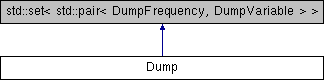JDFTx  1.7.0Dump Class Reference

Stores list of what to output and when, and implements functions to do so. More...

#include <Dump.h>

Inheritance diagram for Dump:## Public Member Functions

void setup (const Everything &)

void operator() (DumpFrequency freq, int iter)

string getFilename (string varName) const
Get the dump filename corresponding to a particular variable name.

bool checkInterval (DumpFrequency freq, int iter) const
Check whether to dump at given frequency and iteration:

## Public Attributes

std::shared_ptr< class DOSdos
density-of-states calculator

std::shared_ptr< struct Polarizabilitypolarizability
electronic polarizability calculator

std::shared_ptr< struct ElectronScatteringelectronScattering
electron-electron scattering calculator

std::vector< std::pair< double, double > > densityErange
energy ranges for energy-resolved density output

std::vector< double > fermiDensityLevels
energies at which to evaluate fermi-dirac derivative

std::shared_ptr< struct SlabEpsilonslabEpsilon
slab dielectric function calculator

std::shared_ptr< struct BulkEpsilonbulkEpsilon
bulk dielectric constant calculator

std::shared_ptr< struct ChargedDefectchargedDefect
charged defect correction calculator

std::shared_ptr< struct BGWparamsbgwParams
parameters for BGW calculation, if any

std::shared_ptr< struct DumpCprimedumpCprime
dC/dk calculator, if needed

bool potentialSubtraction
whether to subtract neutral-atom potentials in Dvac and Dtot output

matrix3< int > Munfold
transformation matrix for band structure unfolding

## Friends

class Phonon

class DefectSupercell

struct CommandDump

struct CommandDumpName

struct CommandDumpInterval

## Detailed Description

Stores list of what to output and when, and implements functions to do so.

## ◆ operator()()

 void Dump::operator() ( DumpFrequency freq, int iter )

Dump all variables that should be dumped at the given DumpFrequency type (End/Gummel/Ionic) at the iter'th iteration at that frequency

The documentation for this class was generated from the following file: